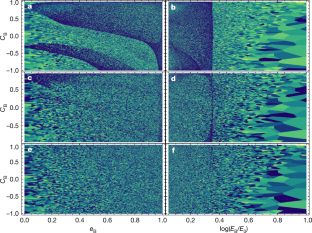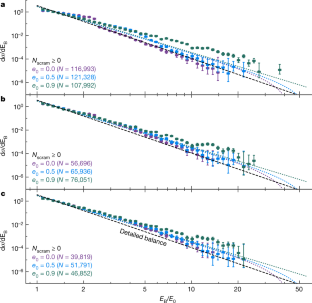# A statistical solution to the chaotic, non-hierarchical three-body problem

## Abstract

The three-body problem is arguably the oldest open question in astrophysics and has resisted a general analytic solution for centuries. Various implementations of perturbation theory provide solutions in portions of parameter space, but only where hierarchies of masses or separations exist. Numerical integrations1 show that bound, non-hierarchical triple systems of Newtonian point particles will almost2 always disintegrate into a single escaping star and a stable bound binary3,4, but the chaotic nature of the three-body problem5 prevents the derivation of tractable6 analytic formulae that deterministically map initial conditions to final outcomes. Chaos, however, also motivates the assumption of ergodicity7,8,9, implying that the distribution of outcomes is uniform across the accessible phase volume. Here we report a statistical solution to the non-hierarchical three-body problem that is derived using the ergodic hypothesis and that provides closed-form distributions of outcomes (for example, binary orbital elements) when given the conserved integrals of motion. We compare our outcome distributions to large ensembles of numerical three-body integrations and find good agreement, so long as we restrict ourselves to ‘resonant’ encounters10 (the roughly 50 per cent of scatterings that undergo chaotic evolution). In analysing our scattering experiments, we identify ‘scrambles’ (periods of time in which no pairwise binaries exist) as the key dynamical state that ergodicizes a non-hierarchical triple system. The generally super-thermal distributions of survivor binary eccentricity that we predict have notable applications to many astrophysical scenarios. For example, non-hierarchical triple systems produced dynamically in globular clusters are a primary formation channel for black-hole mergers11,12,13, but the rates and properties14,15 of the resulting gravitational waves depend on the distribution of post-disintegration eccentricities.

## Access options

from\$8.99

All prices are NET prices.Fig. 2: Topological maps of three-body scattering outcomes for run A.Fig. 3: Marginal distribution of binary energy, dσ/dEB, as a function of dimensionless energy, EB/E0.

## Data availability

The data that support the findings of this study are available from the corresponding author upon reasonable request.

## References

1. 1.

Agekyan, T. A. & Anosova, Z. P. A study of the dynamics of triple systems by means of statistical sampling. Astron. Zh. 44, 1261 (1967).

2. 2.

Šuvakov, M. & Dmitrašinović, V. Three classes of Newtonian three-body planar periodic orbits. Phys. Rev. Lett. 110, 114301 (2013).

3. 3.

Standish, E. M. The dynamical evolution of triple star systems. Astron. Astrophys. 21, 185–191 (1972).

4. 4.

Hut, P. & Bahcall, J. N. Binary–single star scattering. I – numerical experiments for equal masses. Astrophys. J. 268, 319–341 (1983).

5. 5.

Poincaré, H. Les Méthodes Nouvelles de la Mécanique Céleste (Gauthier-Villars et fils, 1892).

6. 6.

Sundman, K. F. Mémoire sur le problème des trois corps. Acta Math. 36, 105–179 (1913).

7. 7.

Fermi, E. High energy nuclear events. Prog. Theor. Phys. 5, 570–583 (1950).

8. 8.

Monaghan, J. J. A statistical theory of the disruption of three-body systems – I. Low angular momentum. Mon. Not. R. Astron. Soc. 176, 63–72 (1976).

9. 9.

Valtonen, M., Mylläri, A., Orlov, V. & Rubinov, A. Dynamics of rotating triple systems: statistical escape theory versus numerical simulations. Mon. Not. R. Astron. Soc. 364, 91–98 (2005).

10. 10.

Heggie, D. C. Binary evolution in stellar dynamics. Mon. Not. R. Astron. Soc. 173, 729–787 (1975).

11. 11.

Portegies Zwart, S. F. & McMillan, S. L. W. Black hole mergers in the Universe. Astrophys. J. Lett. 528, 17–20 (2000).

12. 12.

Rodriguez, C. L., Chatterjee, S. & Rasio, F. A. Binary black hole mergers from globular clusters: masses, merger rates, and the impact of stellar evolution. Phys. Rev. D 93, 084029 (2016).

13. 13.

Hong, J. et al. Binary black hole mergers from globular clusters: the impact of globular cluster properties. Mon. Not. R. Astron. Soc. 480, 5645–5656 (2018).

14. 14.

Samsing, J., MacLeod, M. & Ramirez-Ruiz, E. The formation of eccentric compact binary inspirals and the role of gravitational wave emission in binary–single stellar encounters. Astrophys. J. 784, 71 (2014).

15. 15.

Rodriguez, C. L. et al. Post-Newtonian dynamics in dense star clusters: formation, masses, and merger rates of highly-eccentric black hole binaries. Phys. Rev. D 98, 123005 (2018).

16. 16.

Portegies Zwart, S. F. & Boekholt, T. C. N. Numerical verification of the microscopic time reversibility of Newton’s equations of motion: fighting exponential divergence. Commun. Nonlinear Sci. Numer. Simul. 61, 160–166 (2018).

17. 17.

Hut, P. The topology of three-body scattering. Astron. J. 88, 1549–1559 (1983).

18. 18.

Samsing, J. & Ilan, T. Topology of black hole binary–single interactions. Mon. Not. R. Astron. Soc. 476, 1548–1560 (2018).

19. 19.

Bohr, N. Neutron capture and nuclear constitution. Nature 137, 344–348 (1936).

20. 20.

Monaghan, J. J. A statistical theory of the disruption of three-body systems – II. High angular momentum. Mon. Not. R. Astron. Soc. 177, 583–594 (1976).

21. 21.

Nash, P. E. & Monaghan, J. J. A statistical theory of the disruption of three-body systems – III. Three-dimensional motion. Mon. Not. R. Astron. Soc. 184, 119–125 (1978).

22. 22.

Geller, A. M., Leigh, N. W. C., Giersz, M., Kremer, K. & Rasio, F. A. In search of the thermal eccentricity distribution. Astrophys. J. 872, 165 (2019).

23. 23.

Pomeau, Y. & Manneville, P. Intermittent transition to turbulence in dissipative dynamical systems. Commun. Math. Phys. 74, 189–197 (1980).

24. 24.

Mardling, R. A. & Aarseth, S. J. Tidal interactions in star cluster simulations. Mon. Not. R. Astron. Soc. 321, 398–420 (2001).

25. 25.

Leigh, N. W. C. & Geller, A. M. The dynamical significance of triple star systems in star clusters. Mon. Not. R. Astron. Soc. 432, 2474–2479 (2013).

26. 26.

Leonard, P. J. T. & Fahlman, G. G. On the origin of the blue stragglers in the globular cluster NGC 5053. Astron. J. 102, 994 (1991).

27. 27.

Leigh, N., Sills, A. & Knigge, C. An analytic model for blue straggler formation in globular clusters. Mon. Not. R. Astron. Soc. 416, 1410–1418 (2011).

28. 28.

Ivanova, N. et al. Formation and evolution of compact binaries in globular clusters – I. Binaries with white dwarfs. Mon. Not. R. Astron. Soc. 372, 1043–1059 (2006).

29. 29.

Pooley, D. & Hut, P. Dynamical formation of close binaries in globular clusters: cataclysmic variables. Astrophys. J. Lett. 646, 143–146 (2006).

30. 30.

Ivanova, N., Heinke, C. O., Rasio, F. A., Belczynski, K. & Fregeau, J. M. Formation and evolution of compact binaries in globular clusters – II. Binaries with neutron stars. Mon. Not. R. Astron. Soc. 386, 553–576 (2008).

## Acknowledgements

We acknowledge discussions with D. Heggie, P. Hut, R. Sari and S. Portegies-Zwart, as well as feedback from E. Michaely and O. C. Winter. N.C.S. received financial support from NASA, through Einstein Postdoctoral Fellowship Award number PF5-160145 and the NASA Astrophysics Theory Research Program (grant NNX17AK43G; Principal Investigator, B. Metzger). N.C.S. also thanks the Aspen Center for Physics for its hospitality during early stages of this work. N.W.C.L. acknowledges support by Fondecyt Iniciacion grant number 11180005. We thank the Chinese Academy of Sciences for hosting us as we completed our efforts. We thank M. Valtonen and H. Karttunen, whose book on the three-body problem motivated much of this work.

## Author information

N.C.S. led the analytic work, to which N.W.C.L. contributed significantly. The FEWBODY simulations were performed by N.W.C.L. The comparison between the simulations and the analytic theory was performed jointly by the two authors.

Correspondence to Nicholas C. Stone.

## Ethics declarations

### Competing interests

The authors declare no competing interests.

Peer review information Nature thanks Erez Michaely and Othon Cabo Winter for their contribution to the peer review of this work.

Publisher’s note Springer Nature remains neutral with regard to jurisdictional claims in published maps and institutional affiliations.

## Extended data figures and tables

### Extended Data Fig. 1 Marginal distribution of binary energies, dσ/dEB.

Colours show dimensionless angular momenta $${\tilde{L}}_{0}$$; upper and lower black dashed lines are asymptotic power laws for $${\tilde{L}}_{0}$$ = 1 and $${\tilde{L}}_{0}$$ ≈ 1, respectively. a, Ergodic outcome distributions using the ‘apocentric escape’ (AE) criterion; that is, assuming that disintegration of metastable triples occurs within a strong interaction region of size R = αaB(1 + eB). Here we take α = 2. Solid lines represent equal-mass scattering ensembles (ma = mb = ms) and dotted lines extreme-mass-ratio ensembles (ma = mb = 10ms). b, As in a, but for a ‘simple escape’ (SE) criterion, R = αaB. c, Intermediate-mass-ratio scattering ensembles (ma = mb = 3ms). Solid lines correspond to α = 2 and dotted lines to α = 5. d, As in c, but for ma = mb = 10ms. Note that $${\tilde{L}}_{0}$$ is a dimensionless angular momentum normalized by the circular orbit angular momentum of a binary with energy E0 and masses ma and mb.

### Extended Data Fig. 2 Marginal distribution of binary eccentricity, dσ/deB.

Line styles and assumptions are as in Extended Data Fig. 1, except for the upper and lower black dashed lines, which here show the $${\tilde{L}}_{0}\approx 1$$ and $${\tilde{L}}_{0}\ll 1$$ limits of the dσ/deB distribution, respectively (unlike for dσ/dEB, these limits differ significantly in the AE and SE regimes). In comparable-mass AE calculations, mildly super-thermal outcomes arise from geometric effects when $${\tilde{L}}_{0}\approx 1$$; by contrast, angular-momentum starvation produces extremely super-thermal outcomes when $${\tilde{L}}_{0}\ll 1$$. Small ms values foreclose parts of eB space, as LB ≈ L0.

### Extended Data Fig. 3 Marginal distribution of binary orientation, dσ/dCB.

Assumptions and line styles are as in Extended Data Fig. 1, except that the black dashed lines show (i) an isotropic outcome configuration and (ii) an analytic approximation for dσ/dCB, as labelled in a (for an equal-mass triple with $${\tilde{L}}_{0}=0.5$$). For $${\tilde{L}}_{0}\ll 1$$, surviving binaries are distributed isotropically (as symmetry dictates). Otherwise, binary orientations $${C}_{{\rm{B}}}={\hat{{\bf{L}}}}_{{\rm{B}}}\cdot {\hat{{\bf{L}}}}_{0}$$ are biased towards prograde outcomes. For extreme mass ratios and large $${\tilde{L}}_{0}$$, retrograde outcomes may be entirely prohibited.

## Supplementary information

### Supplementary Information

This Supplementary Information file contains: (1) Chaotic Escape in the Three-Body Problem; (2) Outcomes of Non-Hierarchical Three Body Encounters; (3) Comparison to Numerical Scattering Experiments; (4) Discussion; and associated references.

## Rights and permissions

Reprints and Permissions